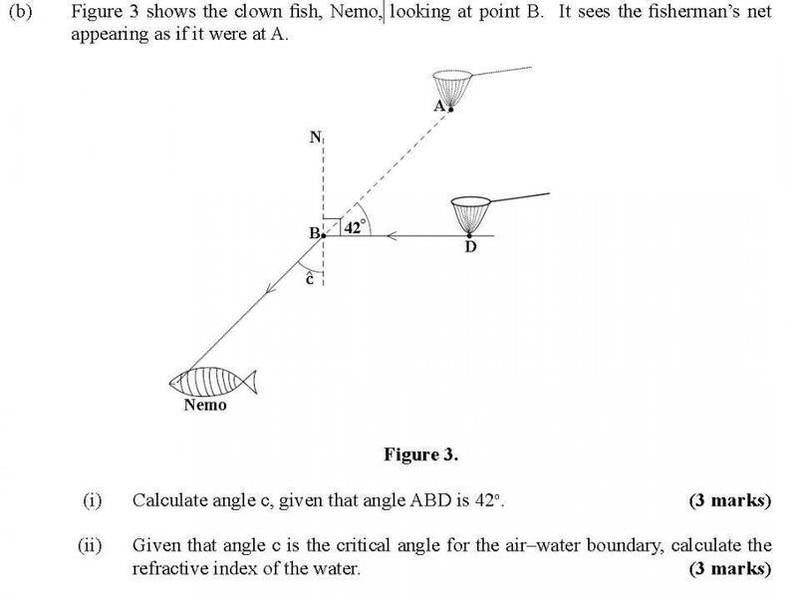# Finding angle of refraction given angle of incidence?

Can someone help me in solving this question ?

my attemp is sin42/sin 90 to get the refractive index then n =sin i/sin r to get c
and for b n=1/sincTSny
Homework Helper
Gold Member
Welcome to PF!

Try to answer part (i) first by examining the diagram and using a little geometry.

•Martin Hanna
Thanks for responding but i am trying but cant figure it out its probably right there in my face but i just cant see it yet

that would be 90 ?

TSny
Homework Helper
Gold Member
Yes, I think that's what you are supposed to assume. The fisherman's net (at D) is assumed to be essentially at the surface of the water.

so there fore the angle of incidence is 90 so that means C would be a critical angle

TSny
Homework Helper
Gold Member
so there fore the angle of incidence is 90 so that means C would be a critical angle
Yes. From the diagram, can you determine the value of the critical angle in degrees?

42 ? i doubt you can just determine it from looking at the diagram as the question asks to calculate it

therefore c must be 180-(42+90)

TSny
Homework Helper
Gold Member
Yes

well it makes sense because the answer is 48 and 48 is the critical angle of air-water boundary so now i find the refractive index 1/48

TSny
Homework Helper
Gold Member
Why 1/48?

because there is a formular which says that N(Refractive index) = 1/ sin C(48) oh i see yeah im suppose to sin the 48

TSny
Homework Helper
Gold Member
Yes, good.

thank you very much